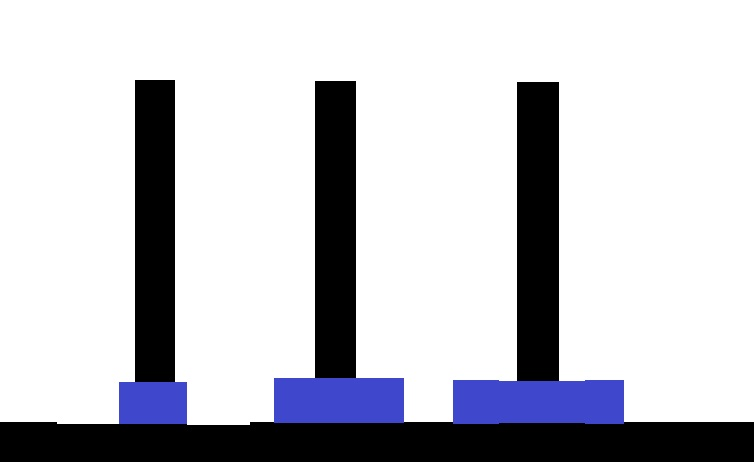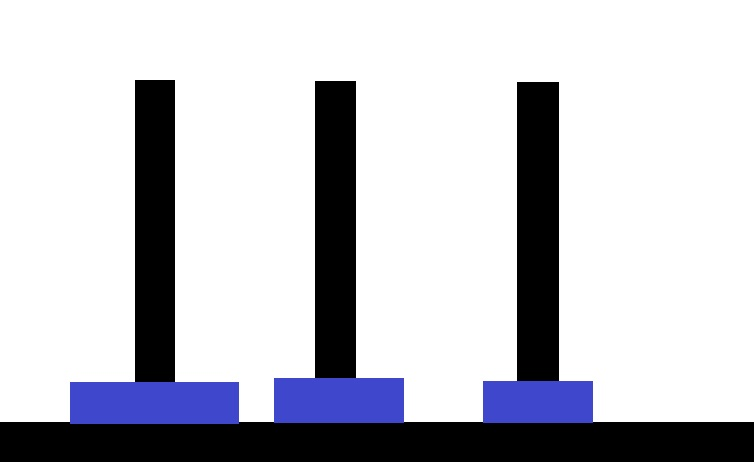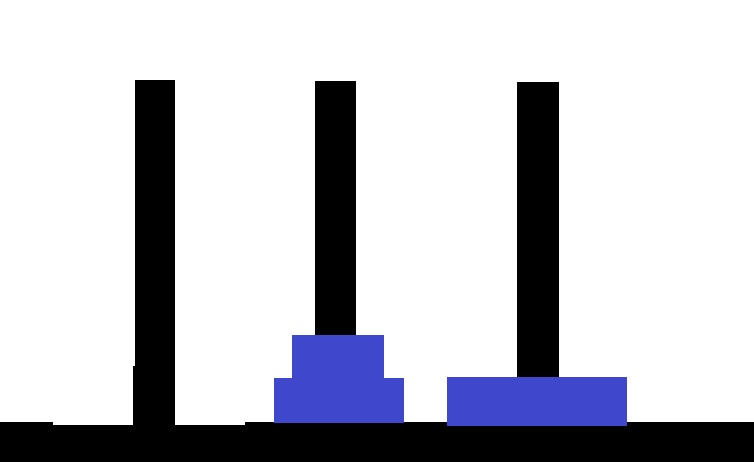### 问题分析• 将 x 轴上的 n-1 个盘子移动到 y 轴上。
• 将 x 轴上最底下的盘子移动到 z 轴上。
• 将 y 轴上的 n-1 个盘子移动到 z 轴上。

### 代码示例

def hanoi(n, x, y, z):
step=0
if n == 1:
print(x, '-->;', z)

else:
hanoi(n - 1, x, z, y)
print(x, '-->', z)
hanoi(n - 1, y, x, z)
n=input("请输入汉诺塔上圆盘的个数:")
n=int(n)
hanoi(n, 'x', 'y', 'z') #调用hanoi函数